Document revision date: 15 July 2002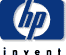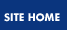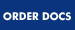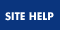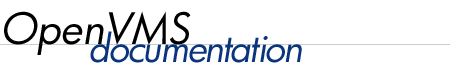## OpenVMS User's Manual

 Previous Contents Index

### 12.6.4 Comparing Character Strings

When you compare two character strings, the strings are compared character by character. Strings of different lengths are not equal (for example, "dogs" is greater than "dog").

The comparison criteria are the ASCII values of the characters. Under these criteria, the digits 0 to 9 are less than the uppercase letters A to Z, and the uppercase letters A to Z are less than the lowercase letters a to z. A character string comparison ends when either of the following conditions is true:

• All the characters have been compared, in which case the strings are equal.
• The first mismatch occurs.

Table 12-1 lists different types of string comparisons.

Table 12-1 String Comparisons
Comparison Operator Description
Equal to .EQS. Compares one character string to another for equality.
Greater than or equal to .GES. Compares one character string to another for greater or equal value in the first specified string.
Greater than .GTS. Compares one character string to another for a greater value in the first specified string.
Less than or equal to .LES. Compares one character string to another for a lesser or equal value in the first specified string.
Less than .LTS. Compares one character string to another for a lesser value in the first specified string.
Not equal .NES. Compares one character string to another for inequality.

In all of the following examples, assume that the symbol LAST_NAME has the value "WHITFIELD".

• In the following example, the symbol TEST_NAME is evaluated as 0 (False); the value of the symbol LAST_NAME does not equal the literal "HILL":

 ```\$ TEST_NAME = LAST_NAME .EQS. "Hill" \$ SHOW SYMBOL TEST_NAME TEST_NAME = 0 ... ```

• In the following example, the symbol TEST_NAME is evaluated as 1 (True); the value of the symbol LAST_NAME is greater than or equal to the literal "HILL":

 ```\$ TEST_NAME = LAST_NAME .GES. "HILL" \$ SHOW SYMBOL TEST_NAME TEST_NAME = 1 ... ```

• In the following example, the symbol TEST_NAME is evaluated as 1 (True); the value of the symbol LAST_NAME is greater than the literal "HILL.":

 ```\$ TEST_NAME = LAST_NAME .GTS. "HILL" \$ SHOW SYMBOL TEST_NAME TEST_NAME = 1 ... ```

• In the following example, the symbol TEST_NAME is evaluated as 0 (False); the value of the symbol LAST_NAME is not less than or equal to the literal "HILL":

 ```\$ TEST_NAME = LAST_NAME .LES. "HILL" \$ SHOW SYMBOL TEST_NAME TEST_NAME = 0 ... ```

• In the following example, the symbol TEST_NAME is evaluated as 0 (False); the value of the symbol LAST_NAME is not less than the literal "HILL":

 ```\$ TEST_NAME = LAST_NAME .LTS. "HILL" \$ SHOW SYMBOL TEST_NAME TEST_NAME = 0 ... ```

• In the following example, the symbol TEST_NAME is evaluated as 1 (True); the value of the symbol LAST_NAME does not equal the literal "HILL":

 ```\$ TEST_NAME = LAST_NAME .NES. "HILL" \$ SHOW SYMBOL TEST_NAME TEST_NAME = 1 ... ```

### 12.6.5 Replacing Substrings

You can replace part of a character string with another character string by specifying the position and size of the replacement string. The format for local symbols is:
 symbol-name[offset,size] := replacement-string

The format for global symbols is:
 symbol-name[offset,size] :== replacement-string

The elements are as follows:
 offset An integer that indicates the position of the replacement string relative to the first character in the original string. An offset of 0 means the first character in the symbol, an offset of 1 means the second character, and so on. size An integer that indicates the length of the replacement string.

To replace substrings, observe the following rules:

• The square brackets are required notation. No spaces are allowed between the symbol name and the left bracket.
• Integer values for size and offset values can range from 0 to 768.
• The replacement string must be a character string.
• The symbol name you specify can be undefined initially. The assignment statement creates the symbol name and if necessary, provides leading or trailing spaces in the symbol value.
• You can specify an offset and size to create a symbol that represents a blank line.

Lining up records in columns makes a list easier to read and sort. You can use this format to specify how you want data to be stored.

In the following example, the first assignment statement gives the symbol A the value PACKRAT. The second statement specifies that MUSK replace the first four characters in the value of A. The result is that the value of A becomes MUSKRAT.

 ```\$ A := PACKRAT \$ A[0,4] := MUSK \$ SHOW SYMBOL A A = "MUSKRAT" ```

In the following example, the symbol B does not have a previous value, so it is given a value of four leading spaces followed by RAT:

 ```\$ B[4,3] := RAT ```

In the following example, a value of 80 blank spaces is assigned to the symbol LINE:

 ```\$ LINE[0,80]:= " " ```

In the following example, the first statement fills in the first 15 columns of DATA with whatever value NAME has. The second statement fills in column 18 with whatever value GRADE has. Columns 16 and 17 contain blanks:

 ```\$ DATA[0,15] := 'NAME' \$ DATA[17,1] := 'GRADE' ```

## 12.7 Using Numeric Values and Expressions

A number can have the following values:

• Decimal --- the ASCII characters 0 to 9
• Hexadecimal --- the ASCII characters 0 to 9 and A to F
• Octal --- the ASCII characters 0 to 7

The number you assign to a symbol must be in the range --2147483648 to 2147483647 (decimal). An error is not reported if a number outside this range is specified or calculated but an incorrect value results.

### 12.7.1 Specifying Numbers

At DCL command level and within command procedures, specify a number as follows:

• Positive numbers
Specify a positive number by typing the appropriate digits.
• Negative numbers
Precede a negative number with a minus sign ( - ).
Specify a number in a radix other than decimal by preceding the number (but not the minus sign) with %X for hexadecimal numbers and %0 for octal numbers.
• Fractions
A number cannot include a decimal point. In calculations, fractions are truncated. For example, 8 divided by 3 equals 2.

In the following example, the number 13 is assigned to the symbol DOG_COUNT:

 ```\$ DOG_COUNT = 13 \$ SHOW SYMBOL DOG_COUNT DOG_COUNT = 13 Hex = 0000000D Octal = 00000000015 ```

In the following example, the negative number ( -15237 ) is represented with a minus sign ( - ):

 ```\$ BALANCE = -15237 \$ SHOW SYMBOL BALANCE BALANCE = -15237 Hex = FFFFC47B Octal = 37777742173 ```

In the following example, the hexadecimal number D is represented with the prefix %X:

 ```\$ DOG_COUNT = %XD \$ SHOW SYMBOL DOG_COUNT DOG_COUNT = 13 Hex = 0000000D Octal = 00000000015 \$ BALANCE = -%X3B85 \$ SHOW SYMBOL BALANCE BALANCE = -15237 Hex = FFFFC47B Octal = 37777742173 ```

### 12.7.2 Internal Storage of Numbers

Numbers are stored internally as signed 4-byte integers, called longwords; positive numbers have values of 0 to 2147483647 and negative numbers have values of 4294967296 minus the absolute value of the number. The number -15237 , for example, is stored as 4294952059. Negative numbers are converted back to minus-sign format for ASCII or decimal displays; however, they are not converted back for hexadecimal and octal displays. For example, the number -15237 appears in displays as hexadecimal FFFFC47B (decimal 4294952059) rather than hexadecimal --00003B85.

Numbers are stored in text files as a series of digits using ASCII conventions (for example, the digit 1 has a storage value of 49).

In a numeric expression, the values involved must be literal numbers (such as 3) or symbols with numeric values. In addition, you can use a character string that represents a number (for example, "23" or " -51 "). If you perform an operation or comparison between a number and a character string, DCL converts the character string to a number.

Numeric expressions combine the following values (called integer operands):

• Integers. For example:

 ```\$ COUNT = 1 ```

• Lexical functions that are evaluated as integers. For example:

 ```\$ B = F\$INTEGER("-9" + 23) ```

• Symbols that have integer values. For example:

 ```\$ A = B - 6 ```

In the preceding example, the symbol B represents the integer value returned by the F\$INTEGER function ( -923 ).

These integer operands can be connected by arithmetic, logical, and comparison operators, as described in the following sections.

### 12.7.3 Performing Arithmetic Operations

You can specify the following arithmetic operations:

• Multiplication
The asterisk (*) multiplies two numbers.
• Division
The slash (/) divides the first specified number by the second specified number. If a number does not divide evenly, the remainder is lost. No rounding takes place.
The plus sign (+) adds two numbers.
• Subtraction
The minus sign (-) subtracts the second specified number from the first specified number.
• Unary plus and minus
The plus and minus signs change the sign of the number they precede.
• The following example demonstrates using multiplication when assigning a symbol:

 ```\$ BALANCE = 142 * 14 \$ SHOW SYMBOL BALANCE BALANCE = 1988 Hex = 000007C4 Octal = 00000003704 ```

• The following example demonstrates using division when assigning a symbol:

 ```\$ BALANCE = BALANCE / 14 \$ SHOW SYMBOL BALANCE BALANCE = 142 Hex = 0000008E Octal = 00000000216 ```

• The following example demonstrates using addition when assigning a symbol:

 ```\$ BALANCE = BALANCE + 37 \$ SHOW SYMBOL BALANCE BALANCE = 179 Hex = 000000B3 Octal = 00000000263 ```

• The following example demonstrates using subtraction when assigning a symbol:

 ```\$ BALANCE = BALANCE - 15416 \$ SHOW SYMBOL BALANCE BALANCE = -15237 Hex = FFFFC47B Octal = 00000142173 ```

• The following example demonstrates using a unary minus sign to change the sign of the number -142:

 ```\$ BALANCE = -(- a142) \$ SHOW SYMBOL BALANCE BALANCE = 142 Hex = 0000008E Octal = 00000000216 ```

### 12.7.4 Comparing Numbers

Table 12-2 lists different types of numeric comparisons.

Table 12-2 Numeric Comparisons
Comparison Operator Description
Equal to .EQ. Compares one number to another for equality.
Greater than or equal to .GE. Compares one number to another for a greater or equal value in the first number.
Greater than .GT. Compares one number to another for a greater value in the first number.
Less than or equal to .LE. Compares one number to another for a lesser or equal value in the first number.
Less than .LT. Compares one number to another for a lesser value in the first number.
Not equal to .NE. Compares one number to another for inequality.

In the following examples, assume that the symbol BALANCE has the value -15237 .

• In the following example, TEST_BALANCE is evaluated as 1 (True); BALANCE equals -15237 :

 ```\$ TEST_BALANCE = BALANCE .EQ. -15237 \$ SHOW SYMBOL TEST_BALANCE TEST_BALANCE = 1 ... ```

• In the following example, TEST_BALANCE is evaluated as 1 (True); BALANCE is greater than or equal to -15237 :

 ```\$ TEST_BALANCE = BALANCE .GE. -15237 \$ SHOW SYMBOL TEST_BALANCE TEST_BALANCE = 1 ... ```

• In the following example, TEST_BALANCE is evaluated as 0 (False); BALANCE is not greater than -15237 :

 ```\$ TEST_BALANCE = BALANCE .GT. -15237 \$ SHOW SYMBOL TEST_BALANCE TEST_BALANCE = 0 ... ```

• In the following example, TEST_BALANCE is evaluated as 1 (True); BALANCE is less than or equal to -15237 :

 ```\$ TEST_BALANCE = BALANCE .LE. -15237 \$ SHOW SYMBOL TEST_BALANCE TEST_BALANCE = 1 ... ```

• In the following example, TEST_BALANCE is evaluated as 0 (False); BALANCE is not less than -15237 :

 ```\$ TEST_BALANCE = BALANCE .LT. -15237 \$ SHOW SYMBOL TEST_BALANCE TEST_BALANCE = 0 ... ```

• In the following example, TEST_BALANCE is evaluated as 0 (False); BALANCE equals -15237 :

 ```\$ TEST_BALANCE = BALANCE .NE. -15237 \$ SHOW SYMBOL TEST_BALANCE TEST_BALANCE = 0 ... ```

### 12.7.5 Performing Numeric Overlays

You can perform binary (bit-level) overlays of the current symbol value by using a special format of the assignment statement. For local symbols, the format is:
 symbol-name[bit-position,size] = replacement-expression

For global symbols, the format is:
 symbol-name[bit-position,size] == replacement-expression

The elements are as follows:
 bit-position An integer that indicates the location relative to bit 0 at which the overlay is to occur. size An integer that indicates the number of bits to be overlaid.

To use numeric overlays, observe the following rules:

• The square brackets ([]) are required notation. No spaces are allowed between the symbol name and the left bracket.
• Literal values are assumed to be decimal.
• The maximum length for size is 32 bits.
• Replacement expression must be a numeric expression.
• When symbol-name is either undefined or defined as a string, the result of the overlay is a string. Otherwise, the result is an integer.

The following example defines the symbol BELL as the value 7. The low-order byte of BELL has the binary value 00000111. By changing the 0 at offset 5 to 1 (beginning with 0, count bits from right to left), you create the binary value 00100111 (decimal value 39):

 ```\$ BELL = 7 \$ BELL[5,1] = 1 \$ SHOW SYMBOL BELL BELL = 39 Hex = 00000027 Octal = 00000000047 ```

## 12.8 Using Logical Values and Expressions

The following sections describe how to use logical values and expressions.

### 12.8.1 Logical Operations

Some operations interpret numbers and character strings as logical data with values as follows:

• True
A number has a logical value of true if it is odd (that is, the low-order bit is 1). A character string has a logical value of true if the first character is an uppercase or lowercase T or Y.
• False
A number has a logical value of false if it is even (that is, the low-order bit is 0). A character string has a logical value of false if the first character is not an uppercase or lowercase T or Y.

In the following examples, DOG_COUNT is assigned the value 13. IF STATUS means if the logical value of STATUS is true.

 ```\$ STATUS = 1 \$ IF STATUS THEN DOG_COUNT = 13 ```

 ```\$ STATUS = "TRUE" \$ IF STATUS THEN DOG_COUNT = 13 ```

### 12.8.2 Logical Expressions

A logical operation affects all the bits in the number being acted upon. The values for logical expressions are integers, and the result of the expression is an integer as well. If you specify a character string value in a logical expression, the string is converted to an integer before the expression is evaluated.

Typically, you use logical expressions to evaluate the low-order bit of a logical value; that is, to determine whether the value is true or false. You can specify the following logical operations:

• .NOT.
The operator .NOT. reverses the bit configuration of a logical value. A true value becomes false and a false value becomes true.
• .AND.
The operator .AND. combines two logical values as follows:
Bit Level Entity Level
1 .AND. 1 = 1 true .AND. true = true
1 .AND. 0 = 0 true .AND. false = false
0 .AND. 1 = 0 false .AND. true = false
0 .AND. 0 = 0 false .AND. false = false
• .OR.
The operator .OR. combines two logical values as follows:
Bit Level Entity Level
1 .OR. 1 = 1 true .OR. true = true
1 .OR. 0 = 1 true .OR. false = true
0 .OR. 1 = 1 false .OR. true = true
0 .OR. 0 = 0 false .OR. false = false

The following example reverses a true value to false. The expression is evaluated as --2; the value is even and is therefore false:

 ```\$ SHOW SYMBOL STATUS STATUS = 1 Hex = 00000001 Octal = 00000000001 \$ STATUS = .NOT. STATUS \$ SHOW SYMBOL STATUS STATUS = -2 Hex = FFFFFFFE Octal = 37777777776 ```

The following example combines a true value and a false value to produce a false value:

 ```\$ STAT1 = "TRUE" \$ STAT2 = "FALSE" \$ STATUS = STAT1 .AND. STAT2 \$ SHOW SYMBOL STATUS STATUS = 0 Hex = 00000000 Octal = 00000000000 ```

The following example combines a true value and a false value to produce a true value:

 ```\$ STAT1 = "TRUE" \$ STAT2 = "FALSE" \$ STATUS = STAT1 .OR. STAT2 \$ SHOW SYMBOL STATUS STATUS = 1 Hex = 00000001 Octal = 00000000001 ```

 Previous Next Contents Index